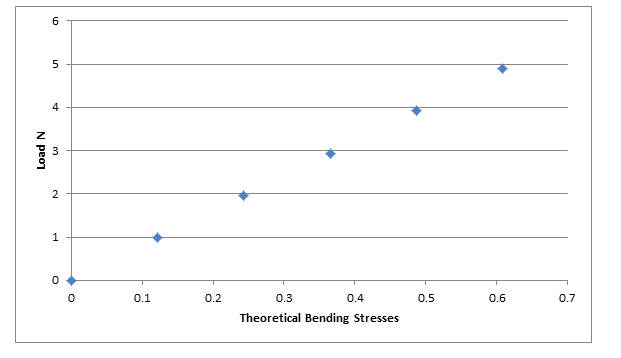# Bending Moment in a Beam Lab Report

## Aim of Bending Moment in a Beam

Aim of this experiment is to study effect of force magnitude on bending moment of beam

## Theory Bending Moment in a Beam

### Beams

A structural element which is designed and used to bear high load of structure and other external load is called beam. There are many different types of beam like cantilever beam, simple supported beam and overhanging beam.

### Bending of Beam

When an external load or the structural load applied in beam is large enough to displace the beam from its present place, then that deflection of beam from its resent axis is called bending of beam.

### Bending Moment

In simple words bending moment is the product of force applied on beam with the distance between the point of application of force and fixed end of the beam

## Introduction to Experiment of Bending Moment in a Beam

This experiment is about studying the effect of force magnitude on bending of beam and for that structure hardware called ‘STR2 bending moment in a beam is used.

According to the figure of STR2 bending moment in beam structure, beam is supported at two points using pivots.

A mechanism is provided which can apply and calculate the force throughout the beam. Free body diagram of the apparatus is shown below.

Figure 1 bending moment apparatusFigure 2 free body diagramIn this experiment load of different magnitude will applied on beam at the same place and bending moment will be calculated using the following formula.

Bending moment = Wa ((l-a))/l

Here
W is a the applied load on beam
a is the distance between the pivot point and point of force application.
l is the total length of the beam

Procedure
The apparatus involve in this experiment use an electronic system intrigued with software to apply load and calculate the bending moment.

Due to which it is very important to follow the steps involve in this experiment in following presented order

Set up the computer software using the guide provided with the apparatus and set it to virtual experiment mode
In property section box, select the option of variable hanger load

From the tool box area take a load of 100 g and replace it with 0 gram at the cut section

Diagram of the force applied and the graph of the resultant force will appear of screen which conform the load replacement.

Software will automatically gather all the data of experiment and save it in memory.

Repeat the third step with 200, 300, 400 and 500 grams and collect the data related to each experiment

Final result provided by software and manually calculation made were compared using graphs

Results

Table 1 bending moment Results
Calculations

Following is the equation which can be used for the bending moment calculation

Bending Moment = W* a*(l-a)/l

Here

W is a the applied load on beam
a is the distance between the pivot point and point of force application.
l is the total length of the beam

For W = 0
Bending Moment = W* a*(l-a)/l=0*400*(580-400)/580=0 N
For W = 0.98
Bending Moment = W* a*(l-a)/l=0.98*400*(580-400)/580=0 N
For W = 1.96
Bending Moment = W* a*(l-a)/l=1.96*400*(580-400)/580=0 N
For W = 2.94
Bending Moment = W* a*(l-a)/l=2.94*400*(580-400)/580=0 N
For W = 3.92
Bending Moment = W* a*(l-a)/l=3.92*400*(580-400)/580=0 N
For W = 4.90
Bending Moment = W* a*(l-a)/l=4.90*400*(580-400)/580=0 N

Percentage error

Percentage Error= PE = (Experimental shear force-Theoretical shear force)/(Theoretical shear force)
× 100%

PE =  (0.5-0.486)/0.486  × 100%

PE =2.88 %

Table 2 bending moment calculationFigure 4 bending moment comparison## Discussion on Bending Moment in a Beam

Values of the bending stresses obtain from the experiment are presented in the table above and they are arranged in the respective cell according to the load that produce that bending moment.

All the data is presented in the graphs and according to that graph the theoretical bending moment is showing linear relation with the load means the value of the theoretical bending moment increase with the increase in the value of applied load and decrease with the decrease in the value of applied load.

The ratio with which there is an increase and decrease in the value of theoretical bending moment is equal to the ratio with which there is an increase and decrease in the value of applied load.

Second graph is between bending stresses and the load but the main purpose of this graph is to compare the theoretical and experimental bending moment.

According there is very little different between both value which show the correctness of apparatus and skills of the worker. Little different between the values is due to human error which cannot be minimize due to the human capabilities limitation.

Conclusion on Bending Moment in a Beam
Aim of this task was to study the effect of different forces on the bending moment in the beam and the result show that there is a linear relationship between bending moment and applied load.

Experimental and theoretical bending moment shows perfect linear relationship with applied load with very little difference in the values of bending moment.